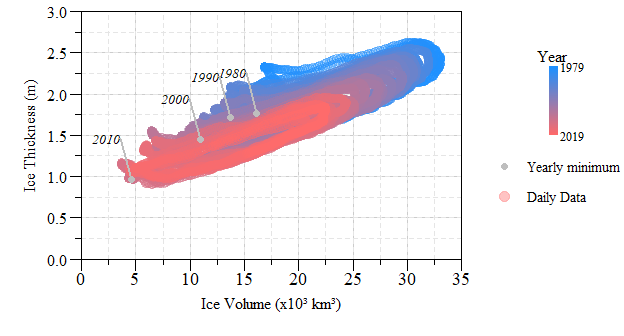# Paul Julian II, PhD

Ecologist, Wetland Biogeochemist, Data-scientist, lover of Rstats.

# July 9, 2019 Eco DataViz

Written on July 9, 2019

Keywords: dataviz, R, Sea Ice

Following the progression of my data viz journey, I decided to tackle some Arctic sea-ice data after checking out Zack Labe’s Arctic Ice figures. The data this week is modeled sea-ice volume and thickness from the Polar Science Center Pan-Arctic Ice Ocean Modeling and Assimilation System (PIOMAS). Sea ice volume is an important climate indicator. It depends on both ice thickness and extent and therefore more directly tied to climate forcing than extent alone.

Each data viz endeavor I try to learn something new or explore existing technique. Dates in R can be stressful to say the least. For anyone who has worked with time-series data would agree. Dates can be formatted as a date format using as.Date(), format(), as.POSIXct() or as.POSIXlt()…most of my time in R is spent formatting dates. Here is a useful page on working with dates in R. The PIOMAS data has three variables…Year, Day of Year (1 - 365) and Thickness (or Volume). I downloaded the data from webpage and unzipped the gzipped tar file using a third party to extract the data, but this can also be done in R. The two data sets volume and thickness data s ASCII files.

#Libraries
#devtools::install_github("SwampThingPaul/AnalystHelper")
library(AnalystHelper);
library(plyr)
library(reshape)
thick.dat=read.table("PIOMAS.thick.daily.1979.2019.Current.v2.1.dat",
header=F,skip=1,col.names=c("Year","Day","Thickness_m"))
head(thick.dat,5L)
##   Year Day Thickness_m
## 1 1979   1       1.951
## 2 1979   2       1.955
## 3 1979   3       1.962
## 4 1979   4       1.965
## 5 1979   5       1.973

The sea-ice volume data is in the same format.

vol.dat=read.table("PIOMAS.vol.daily.1979.2019.Current.v2.1.dat",
vol.dat$Vol_km3=vol.dat$Vol_km3*1E+3;#To convert data 
head(vol.dat,5L)
##   Year Day Vol_km3
## 1 1979   1   26405
## 2 1979   2   26496
## 3 1979   3   26582
## 4 1979   4   26672
## 5 1979   5   26770

The sea-ice thickness data are expressed in meters, and volume in x103 km3. Understanding what the data represent and how they are derived is most of the job of a scientist especially in data visualization. Inherently all data has its limits.

Currently we have two different data files vol.dat and thick.dat, lets get them into one single data.frame and sort the data accordingly (just in case).

dat=merge(thick.dat,vol.dat,c("Year","Day"))
dat=dat[order(dat$Year,dat$Day),]

Alright here come the fun part…dates in R. Remember the data is Year and Day of Year, which mean no month or day (i.e. Date). Essentially you have to back calculate day of the year to an actual date. Thankfully this is pretty easy. Check out ?strptime and ?format!!

dat$month.day=format(strptime(dat$Day,"%j"),"%m-%d")

This gets us Month-Day from day of the year. Now for some tricky. Lets actually make this a date by using paste and leveraging date.fun() from AnalystHelper.

dat$Date=with(dat,date.fun(paste(Year,month.day,sep="-"),tz="GMT")) Viola!! We have a POSIXct formatted field that has Year-Month-Day…in-case you wanted to check the sea-ice volume on your birthday, wedding anniversary, etc. …no one? Just me? …OK moving on!! Some more tricky which comes in handy when aggregating data is to determine the month and year (for monthly summary statistics). Also we can determine what decade the data is from, it wasn’t used in this analysis but something interesting I discovered in my data musings. dat$month.yr=with(dat,date.fun(paste(Year,format(Date,"%m"),01,sep="-"),tz="GMT"))
dat$decade=((dat$Year)%/%10)*10

Now that we have the data put together lets start plotting.

Here we have just daily (modeled) sea-ice thickness data from PIOMAS.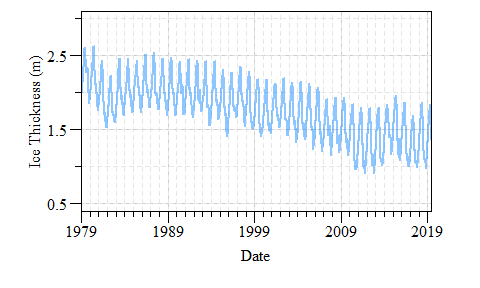Pan Arctic Sea-Ice thickness from 1979 to present. Data source: Polar Science Center - (PIOMAS).

Now we can estimate annual mean and some confidence interval around the mean…lets say 95%.

#Calculate annual mean, sd and N. Excluding 2019 (partial year)
period.mean=ddply(subset(dat,Year!=2019),"Year",summarise,
mean.val=mean(Thickness_m,na.rm=T),
sd.val=sd(Thickness_m,na.rm=T),
N.val=N(Thickness_m))
#Degrees of freedom
period.mean$Df=period.mean$N.val-1
#Student-T statistic
period.mean$Tp=abs(qt(1-0.95,period.mean$Df))
#Lower and Upper CI calculation
period.mean$LCI=with(period.mean,mean.val-sd.val*(Tp/sqrt(N.val))) period.mean$UCI=with(period.mean,mean.val+sd.val*(Tp/sqrt(N.val)))

Now lets add that to the plot with some additional trickery to plot annual mean $$\pm$$ 95% CI stating on Jan 1st of every year.

with(period.mean,lines(date.fun(paste(Year,"01-01",sep="-"),tz="GMT"),mean.val,lty=1,col="red"))
with(period.mean,lines(date.fun(paste(Year,"01-01",sep="-"),tz="GMT"),LCI,lty=2,col="red"))
with(period.mean,lines(date.fun(paste(Year,"01-01",sep="-"),tz="GMT"),UCI,lty=2,col="red"))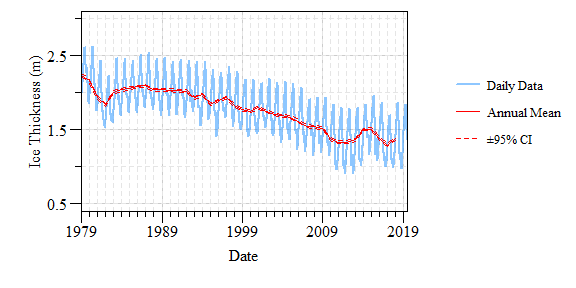Pan Arctic Sea-Ice thickness from 1979 to present with annual mean and 95% confidence interval. Data source: Polar Science Center - (PIOMAS).

What does sea-ice volume look like?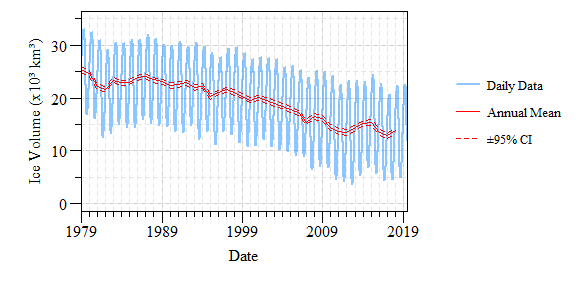Pan Arctic Sea-Ice volume from 1979 to present with annual mean and 95% confidence interval. Data source: Polar Science Center - (PIOMAS).

Some interesting and alarming trends in both thickness and volume for sure! There is an obvious seasonal trend in the data…one way to look at this is to look at the period of record daily change.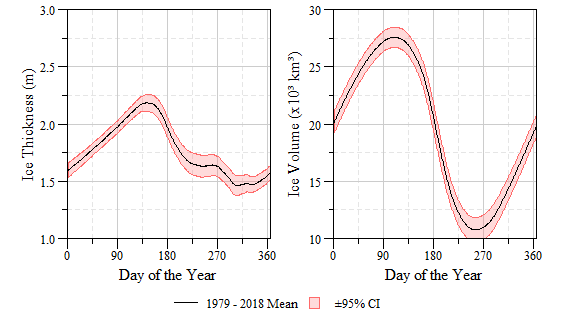Period of record mean (1979 - 2018) daily mean and 95% confidence interval sea-ice volume and thickness. Data source: Polar Science Center - (PIOMAS).

Now how does the thickness versus volume relationship look? Since the volume of data is so much we can do some interesting color coding for the different years. Here I use a color ramp colorRampPalette(c("dodgerblue1","indianred1")) with each year getting a color along the color ramp.

Here is how I set up the color ramp.

N.yrs=length(unique(dat\$Year))
cols=colorRampPalette(c("dodgerblue1","indianred1"))(N.yrs)

In the plot I use a loop to plot each year with a different color.

plot(...)

for(i in 1:N.yrs){
with(subset(dat,Year==yrs.val[i]),
points(Vol_km3,Thickness_m,pch=21,
lwd=0.1,cex=1.25))
}

As is with most data viz, especially in base R is some degree of tricking and layering. To build the color ramp legend I used the following (I adapted a version of this.).

# A raster of the color ramp
legend_image=as.raster(matrix(cols,ncol=1))
# Empty plot
plot(c(0,1),c(0,1),type = 'n', axes = F,xlab = '', ylab = '')
text(0.25+(0.5-0.25)/2,0.85,"Year",xpd=NA)